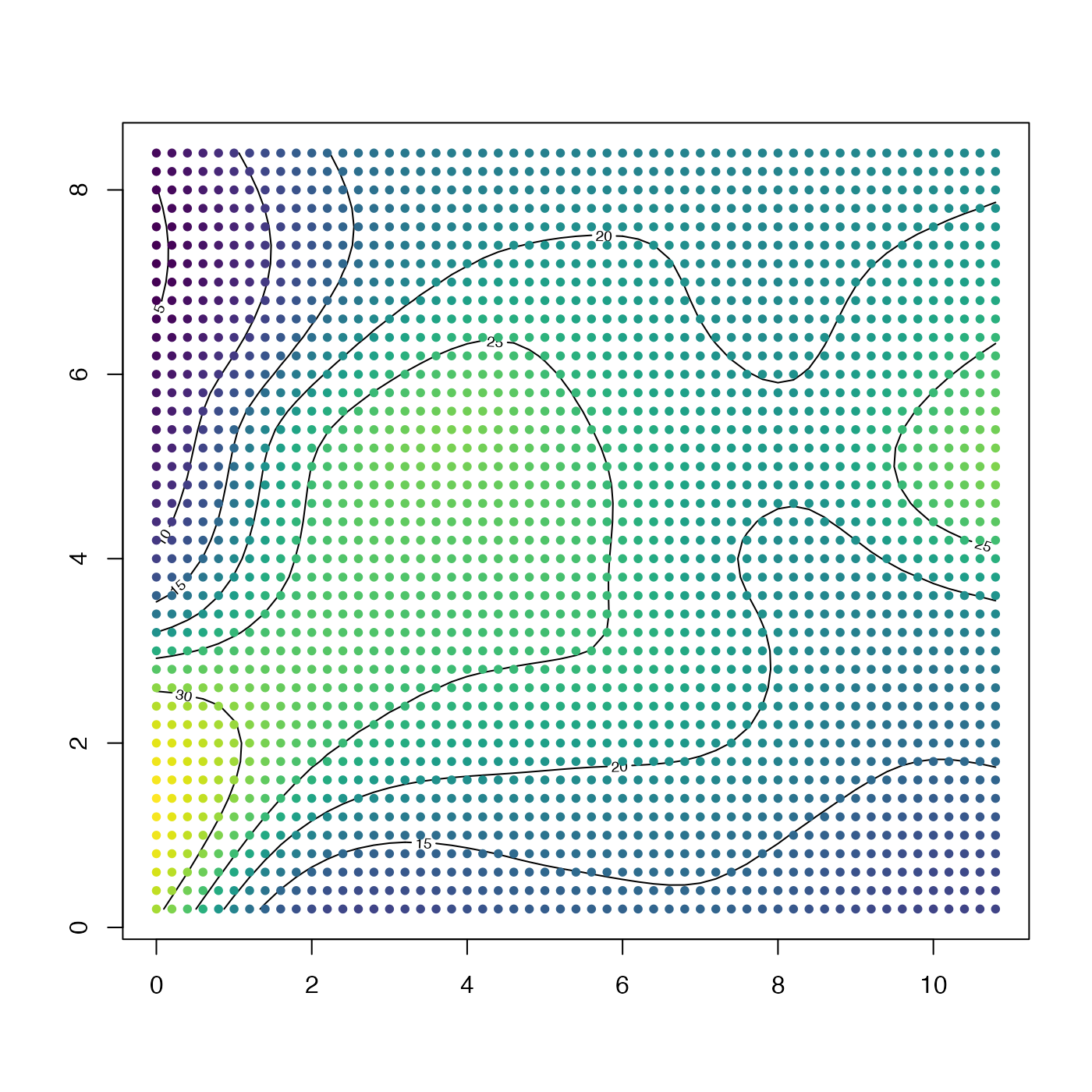Extract the grid points from a grid, returning columns. This is useful for e.g. gridding large datasets, in which the first step might be to use binMean2D(), followed by interpBarnes().

ungrid(x, y, grid)

## Arguments

x a vector holding the x coordinates of grid. a vector holding the y coordinates of grid. a matrix holding the grid.

## Value

A list containing three vectors: x, the grid x values, y, the grid y values, and grid, the grid values.

## Examples

library(oce)
data(wind)
u <- interpBarnes(wind$x, wind$y, wind$z) contour(u$xg, u$yg, u$zg)U <- ungrid(u$xg, u$yg, u$zg) points(U$x, U$y, col=oce.colorsJet(100)[rescale(U$grid, rlow=1, rhigh=100)], pch=20)